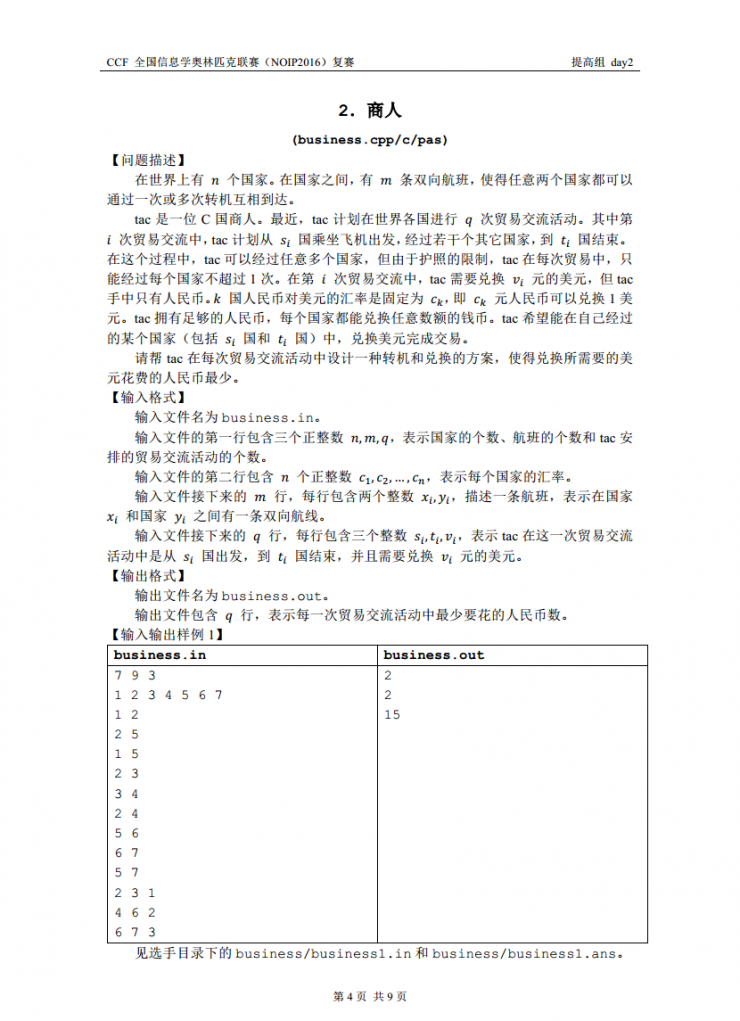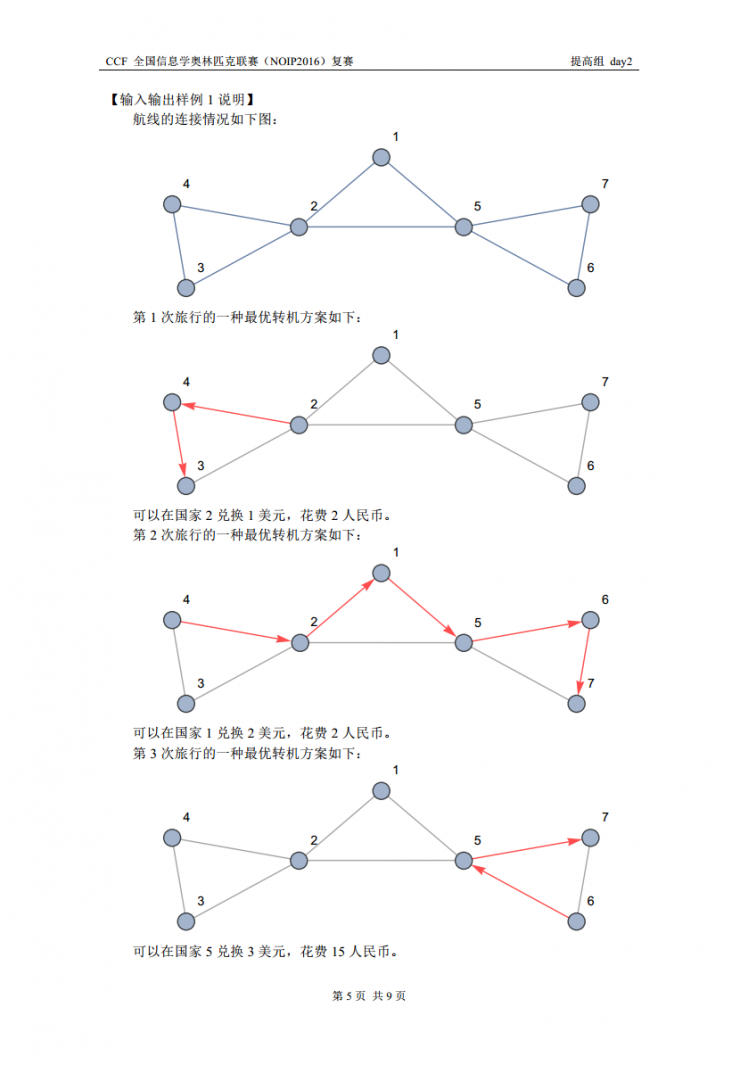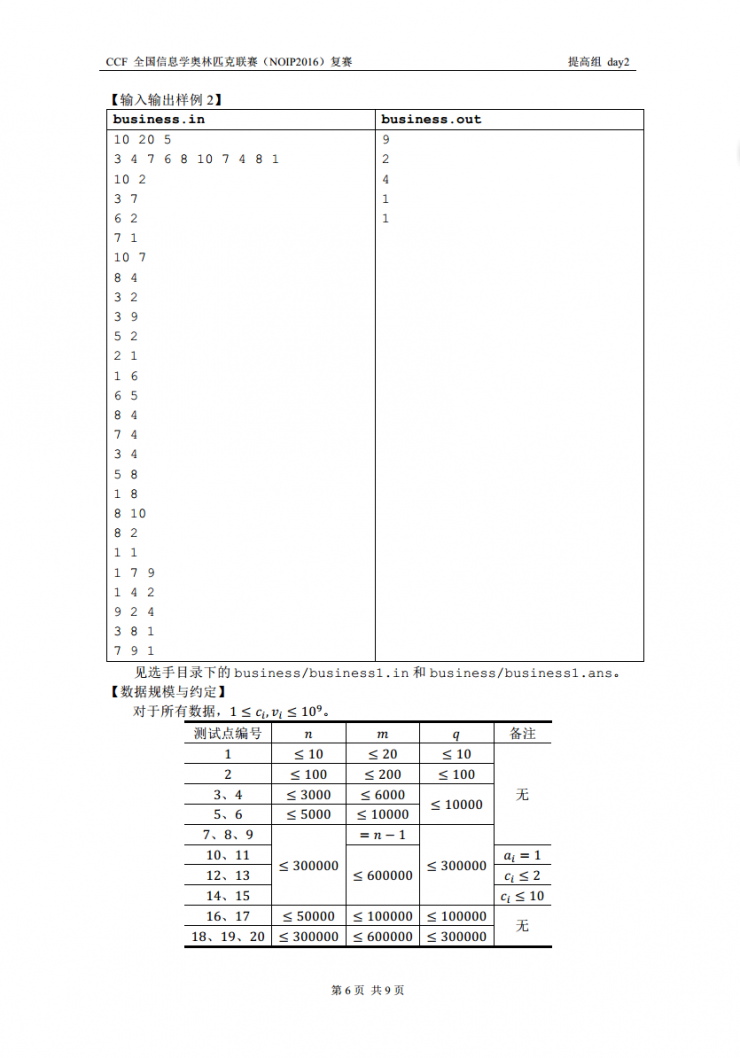…

# YZOJ P2791 商人# YZOJ P3195 [NOI2017]游戏

• ### 题目描述

$$n$$ 场游戏的地图可以用一个小写字母组成的字符串描述。例如：$$S=\underline{\mathrm{xaabxcbc}}$$ 表示小 L 计划进行 $$8$$ 场游戏，其中第 $$1$$ 场和第 $$5$$ 场的地图类型是 $$x$$，适合所有赛车，第 $$2$$ 场和第 $$3$$ 场的地图是 $$a$$，不适合赛车 $$A$$，第 $$4$$ 场和第 $$7$$ 场的地图是 $$b$$，不适合赛车 $$B$$，第 $$6$$ 场和第 $$8$$ 场的地图是 $$c$$，不适合赛车 $$C$$ 。

• ### 输入格式

$$n,d,S$$ 的含义见题目描述，其中 $$S$$ 包含 $$n$$ 个字符，且其中恰好 $$d$$ 个为小写字母 $$x$$ 。

…

# YZOJ P2443 回文子序列

• ### 样例说明

“”（空串）、”a”、”aa”、”aka”、”f”、”ff”、”k”、”kak”、”kk”、”t”

…

# YZOJ P3897 Sevenk Love Oimaster

• ### 数据规模与约定

$$n \leq 10000, q \leq 60000$$ 。

Source: BZOJ 2780 && SPOJ 8093…

…